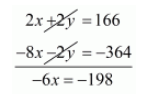Deepak Scored 45->99%ile with Bounce Back Crack Course. You can do it too!

# In a cyclic quadrilateral ABCD, ∠A = (2x + 4)°, ∠B = (y + 3)°, ∠C = (2y + 10)°,

Question:

In a cyclic quadrilateral $A B C D, \angle A=(2 x+4)^{\circ}, \angle B=(y+3)^{\circ}, \angle C=(2 y+10)^{\circ}, \angle D=(4 x-5)^{\circ}$. Find the four angles.

Solution:

We know that the sum of the opposite angles of cyclic quadrilateral is $180^{\circ}$ in the cyclic quadrilateral $A B C D$, angles $A$ and $C$ and angles $B$ and $D$ pairs of opposite angles

Therefore $\angle A+\angle C=180^{\circ}$ and $\angle B+\angle D=180^{\circ}$

Taking $\angle A+\angle C=180^{\circ}$

By substituting $\angle A=(2 x+4)^{\circ}$ and $\angle c=(2 y+10)^{\circ}$ we get

$2 x+4+2 y+10=180$

$2 x+2 y+14=180^{\circ}$

$2 x+2 y=180^{\circ}-14^{\circ}$

$2 x+2 y=166 \cdots(i)$

Taking $\angle B+\angle D=180^{\circ}$

By substituting $\angle B=(y+3)^{\circ}$ and $\angle D=(4 x-5)^{\circ}$ we get

$y+3+4 x-5=180^{\circ}$

$4 x+y-5+3=180^{\circ}$

$4 x+y-2=180^{\circ}$

$4 x+y=180^{\circ}+2^{\circ}$

$4 x+y=182^{\circ} \cdots(i i)$

By multiplying equation $($ ii $)$ by 2 we get $8 x+2 y=364 \cdots($ iii $)$

By subtracting equation $(i i i)$ from $(i)$ we get$x=\frac{-198}{-6}$

$x=33^{\circ}$

By substituting $x=33^{\circ}$ in equation $(i i)$ we get

$4 x+y=182$

$4 \times 33+y=182$

$132+y=182$

$y=182-132$

$y=50$

The angles of a cyclic quadrilateral are

$\angle A=2 x+4$

$=2 \times 33+4$

$=66+4$

$=70^{\circ}$

$\angle B=y+3$

$=50+3$

$=53^{\circ}$

$\angle C=2 y+10^{\circ}$

$=2 \times 50+10$

$=100+10$

$=110^{\circ}$

$\angle D=4 x-5$

$=4 \times 33-5$

$=132-5$

$=127^{\circ}$

Hence, the angles of cyclic quadrilateral $\mathrm{ABCD}$ are $\angle A=70^{\circ}, \angle B=53^{\circ}, \angle C=110^{\circ}, \angle D=127^{\circ}$